Open in App
Not now

# Class 8 NCERT Solutions – Chapter 15 Introduction to Graphs – Exercise 15.3

• Last Updated : 20 Nov, 2020

### Question 1: Draw the graphs for the following tables of values, with suitable scales on the axes.

(a) Cost of apples

(b) Distance travelled by car

(i) How much distance did the car cover during the period 7.30 a.m. to 8 a.m.?

(ii) What was the time when the car had covered a distance of 100 km since it’s start?

(c) Interest on deposits for a year.

(i) Does the graph pass through the origin?

(ii) Use the graph to find the interest on Rs 2500 for a year.

(iii) To get an interest of Rs. 280 per year, how much money should be deposited?

Solution:

(a) Represent the “Number of apples” as X-axis and “Cost in Rs” as Y-axis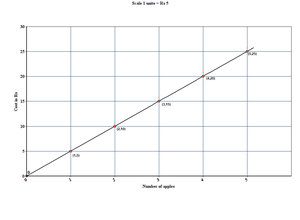(b) Represent the “Times (in hrs)” as X-axis and “Distance (in km)” as Y-axis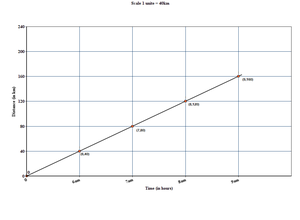(i) The car covered 20 km distance during the period 7.30 a.m. to 8 a.m.

(ii) It was 7.30 am, when the car covered a distance of 100 km since it’s start.

(c) Represent the “Simple Interest (in Rs)” on x-axis and “Deposit (in Rs)” on y-axis.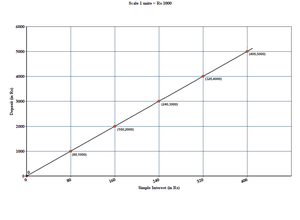(i) Yes, the graph passes through origin.

(ii) The interest on Rs. 2500 is Rs. 200 for a year.

(iii) Rs. 3500 should be deposited for interest of Rs. 280 per year.

### Question 2: Draw a graph for the following

(i)

Is it linear graph?

(ii)

Is it linear graph?

Solution:

(i) Represent the “Side of square(in cm)” on x-axis and “Perimeter(in cm)” on y-axis.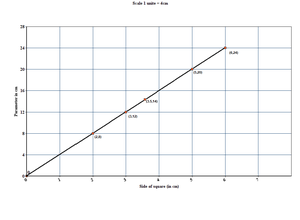Yes, the graph is a linear graph.

(ii) Represent the “Side of square(in cm)” on x-axis and “Area(in cm2)” on y-axis.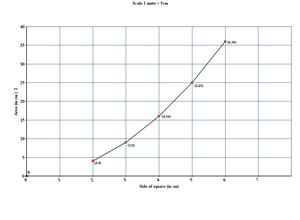No, the graph is not a linear graph because the graph does not provide a straight line.

My Personal Notes arrow_drop_up
Related Articles